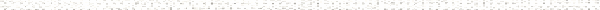A Precalculus Outline

 Chapter 6:  Exponential and Logarithmic FunctionsObjective: Graphing Exponential FunctionsObjective: Graphing Logarithm FunctionsObjective: Common and Natural Logarithmic FunctionsObjective: Find the domain of logarithmic functionsObjective: Converting between exponential and logarithmic formObjective: Evaluating LogarithmsObjective: Finding domain algebraicallyObjective: Sign diagramObjective: Quadratic inequalitiesObjective: Polynomial inequalitiesObjective: Rational inequalitiesObjective: Graphing functionsObjective: Inverse functionObjective: One-to-oneObjective: Horizontal line testObjective: Graphs of inverse functionsObjective: Restricting a function to make it one-to-oneObjective: Understanding the Power Rule of LogarithmsObjective: Understanding the Product Rule of LogarithmsObjective: Understanding the Quotient Rule of LogarithmsObjective: Understanding the Change of Base Formula for LogarithmsObjective: Using Properties of LogarithmsObjective: Properties of exponentsObjective: Logarithm functionObjective: Common logarithmsObjective: Natural logarithmsObjective: Solving equations involving exponential functionsObjective: Properties of exponential functionsObjective: Properties of logarithmsObjective: Sign diagramObjective: Solving equations involving logarithm functionsObjective: Properties of exponential functionsObjective: Properties of logarithmsObjective: Sign diagramObjective: Understanding continuously compounded interest formulaObjective: Understanding the compound interest formulaObjective: Understanding the Exponential Growth and Decay formulaObjective: Solving Exponential Growth ApplicationsObjective: Solving Radioactive Decay ApplicationsObjective: Newton's Law of CoolingObjective: Introduction to Simple and Compound InterestObjective: Solving Compound Interest ProblemsObjective: Graphing exponential functionsObjective: Graphing logarithm functionsObjective: Solving equations involving exponential functionsObjective: Solving equations involving logarithms